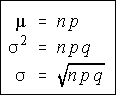###Author Topic: Binomial Probabilities  (Read 124 times)

0 Members and 1 Guest are viewing this topic.

#### kav

• Hero Member
••• Posts: 2367
• Thanked: 1327 times
• Gender:##### Binomial Probabilities
« on: September 14, 2019, 01:13:35 AM »
Binomial Probability Function Example: What is the probability of rolling exactly two sixes in 6 rolls of a die?
There are five things you need to do to work a binomial story problem.
• Define Success first.  Success must be for a single trial. Success = "Rolling a 6 on a single die"
• Define the probability of success (p): p = 1/6
• Find the probability of failure: q = 5/6
• Define the number of trials: n = 6
• Define the number of successes out of those trials: x = 2
Anytime a six appears, it is a success (denoted S) and anytime something else appears, it is a failure (denoted F).  The ways you can get exactly 2 successes in 6 trials are given below.  The probability of each is written to the right of the way it could occur.  Because the trials are independent, the probability of the event (all six dice) is the product of each probability of each outcome (die)
` 1 FFFFSS  5/6 * 5/6 * 5/6 * 5/6 * 1/6 * 1/6 = (1/6)^2 * (5/6)^4 2 FFFSFS  5/6 * 5/6 * 5/6 * 1/6 * 5/6 * 1/6 = (1/6)^2 * (5/6)^4 3 FFFSSF  5/6 * 5/6 * 5/6 * 1/6 * 1/6 * 5/6 = (1/6)^2 * (5/6)^4 4 FFSFFS  5/6 * 5/6 * 1/6 * 5/6 * 5/6 * 1/6 = (1/6)^2 * (5/6)^4 5 FFSFSF  5/6 * 5/6 * 1/6 * 5/6 * 1/6 * 5/6 = (1/6)^2 * (5/6)^4 6 FFSSFF  5/6 * 5/6 * 1/6 * 1/6 * 5/6 * 5/6 = (1/6)^2 * (5/6)^4 7 FSFFFS  5/6 * 1/6 * 5/6 * 5/6 * 5/6 * 1/6 = (1/6)^2 * (5/6)^4 8 FSFFSF  5/6 * 1/6 * 5/6 * 5/6 * 1/6 * 5/6 = (1/6)^2 * (5/6)^4 9 FSFSFF  5/6 * 1/6 * 5/6 * 1/6 * 5/6 * 5/6 = (1/6)^2 * (5/6)^410 FSSFFF  5/6 * 1/6 * 1/6 * 5/6 * 5/6 * 5/6 = (1/6)^2 * (5/6)^411 SFFFFS  1/6 * 5/6 * 5/6 * 5/6 * 5/6 * 1/6 = (1/6)^2 * (5/6)^412 SFFFSF  1/6 * 5/6 * 5/6 * 5/6 * 1/6 * 5/6 = (1/6)^2 * (5/6)^413 SFFSFF  1/6 * 5/6 * 5/6 * 1/6 * 5/6 * 5/6 = (1/6)^2 * (5/6)^414 SFSFFF  1/6 * 5/6 * 1/6 * 5/6 * 5/6 * 5/6 = (1/6)^2 * (5/6)^415 SSFFFF  1/6 * 1/6 * 5/6 * 5/6 * 5/6 * 5/6 = (1/6)^2 * (5/6)^4`

Notice that each of the 15 probabilities are exactly the same: (1/6)^2 * (5/6)^4.
Also, note that the 1/6 is the probability of success and you needed 2 successes.  The 5/6 is the probability of failure, and if 2 of the 6 trials were success, then 4 of the 6 must be failures.  Note that 2 is the value of x and 4 is the value of n-x.
Further note that there are fifteen ways this can occur.  This is the number of ways 2 successes can be occur in 6 trials without repetition and order not being important, or a combination of 6 things, 2 at a time.
The probability of getting exactly x success in n trials, with the probability of success on a single trial being p is:
`   P(X=x) = nCx * p^x * q^(n-x) `
Example: A coin is tossed 10 times.  What is the probability that exactly 6 heads will occur.
• Success = "A head is flipped on a single coin"
• p = 0.5
• q = 0.5
• n = 10
• x = 6
P(x=6) = 10C6 * 0.5^6 * 0.5^4 = 210 * 0.015625 * 0.0625 = 0.205078125

Mean, Variance, and Standard Deviation
The mean, variance, and standard deviation of a binomial distribution are extremely easy to find.Another way to remember the variance is mu-q (since the np is mu). Example: Find the mean, variance, and standard deviation for the number of sixes that appear when rolling 30 dice.
Success = "a six is rolled on a single die".  p = 1/6, q = 5/6.
The mean is 30 * (1/6) = 5.  The variance is 30 * (1/6) * (5/6) = 25/6.  The standard deviation is the square root of the variance = 2.041241452 (approx)